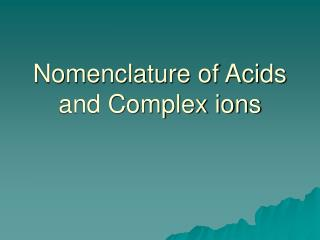DownloadDownload PresentationNomenclature of Acids and Complex ions

Nomenclature of Acids and Complex ions

Télécharger la présentationNomenclature of Acids and Complex ions

- - - - - - - - - - - - - - - - - - - - - - - - - - - E N D - - - - - - - - - - - - - - - - - - - - - - - - - - -
Presentation Transcript

1. Nomenclature of Acids and Complex ions

2. Coordination Compounds • Derived from two simpler compounds joined together. • A Complex consists of a central atom (usually a metal) and attached groups called ligands. Examples: [Co(NH3)6]Cl3 and [CoCl(NH3)5]Cl2

3. Practice • What is the charge of the cation and the metal in [CoCl(NH3)5]Cl2 ? There are two Cl- so the total neg. charge = 2- so thetotal positive charge must be 2+ Therefore, [CoCl(NH3)] 2+ The charge on Co is 2+, since Cl is 1- and NH3 is 0

4. Complex ions • Al(H2O)63+ Only one lone pair is shown on each water molecule. The other lone pair is pointing away from the aluminum and so isn't involved in the bonding. The resulting ion looks like this.

5. Bonding in Complexes • A complex ion has a metal ion at its center with a number of other molecules or ions attached by coordinate covalent or dative bonds. • A dative bond is a covalent bond in which both electrons come from the same atom.

6. The reaction between ammonia and hydrogen chloride

7. Dissolving hydrogen chloride in water to make hydrochloric acid • A hydrogen ion (H+) is transferred from the chlorine to one of the lone pairs on the oxygen atom forming a dative bond. • The H3O+ ion is called the hydronium ion and is usually represented as H+.

8. Nomenclature of Acids • Bianary Acids – compounds of H+ and a non-metal • Use the prefix hydro and suffix –ic and add acid Example: HCl – hydrochloricacid HBr – hydrobromic acid

9. Oxoacids • Contain H and O and another element • No prefix hydro • For ions ending in ate add the suffix –ic and the word acid Example: H2SO4 – (sulfate ion) sulfuricacid HNO3 – (nitrate ion) nitricacid HClO3 – (chlorate ion) choricacid

10. Oxoacids • For ions ending in ite add the suffix –ous and acid Examples: H2SO3 – (sulfite ion) sulfurousacid HNO2 – nitrousacid HClO2 – chlorousacid

11. Oxoacids • For acids with one less oxygen than the –ite ion use the prefix hypo • For acids with one more oxygen than the –ate ion use the prefix per Example ClO2 is chlorite, so ClO is hypochlorite ClO3 is chlorate, so ClO4 is perchlorate

12. Oxoacids • HClO is called? • Hypochorous acid • HClO4 is called? • Perchloric acid • H3PO4 is called? • Phosporic acid

13. Summary of Oxoacids Anion Acid Name ClO- (hypochlorite) hypochlorous acid ClO2 - (chlorite) chlorous acid ClO3 - (chlorate) chloric acid ClO4 - (perchlorate) perchloric acid